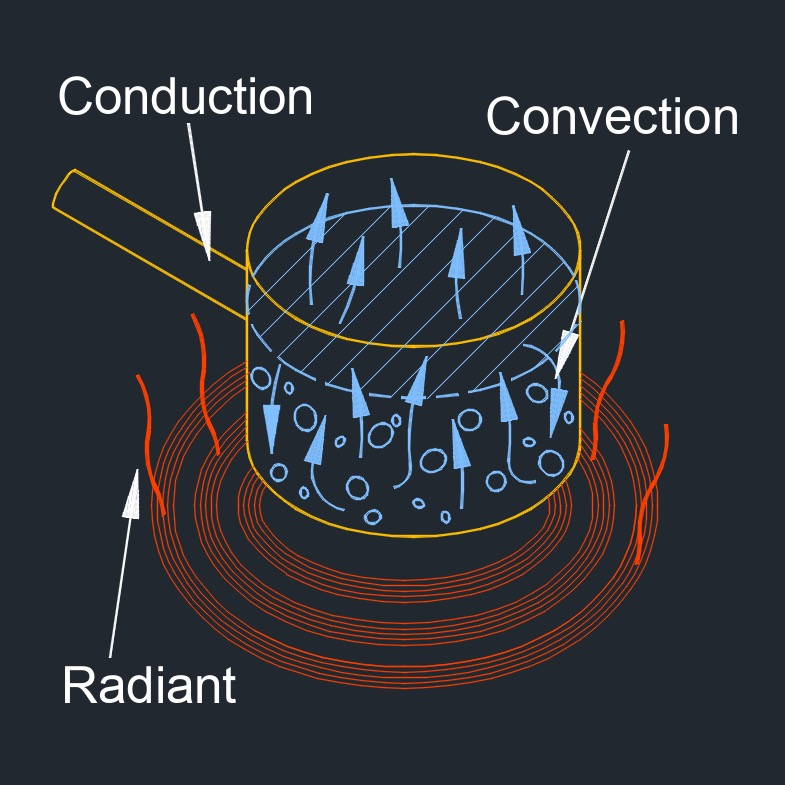# Thermal Energy

on . Posted in ThermodynamicsThermal energy, abbreviated as Q, also known as heat energy and heat transfer, is the exertion of power that is created by heat, or the increase in temperature.  Thermal energy refers to the internal energy of a system due to the random motion of its particles.  It is a form of kinetic energy associated with the microscopic motion of atoms and molecules within a substance.  The temperature of a substance is a measure of the average kinetic energy of its particles.  As the temperature increases, the particles move more rapidly and their kinetic energy increases, resulting in higher thermal energy.

Thermal energy can be transferred between objects or systems through processes such as conduction, convection, and radiation.  When two objects at different temperatures come into contact, heat energy flows from the object with higher temperature to the one with lower temperature, until thermal equilibrium is reached.

Thermal energy plays a fundamental role in many everyday phenomena and scientific disciplines.  It is involved in processes such as heating and cooling, phase changes (such as melting and evaporation), and the behavior of gases.  It is also harnessed for various applications, including power generation, heating systems, and refrigeration.

## Thermal Energy formula

$$\large{ Q = m \; c \; \Delta T }$$
Symbol English Metric
$$\large{ Q }$$ = thermal energy $$\large{Btu}$$ $$\large{\frac{kg-m^2}{s^2}}$$
$$\large{ m }$$ = mass $$\large{ lbm }$$ $$\large{ kg }$$
$$\large{ c }$$ = specific heat $$\large{\frac{Btu}{lbm-F}}$$ $$\large{\frac{kJ}{kg-K}}$$
$$\large{ \Delta T }$$ = temperature differential $$\large{ F }$$ $$\large{ K }$$